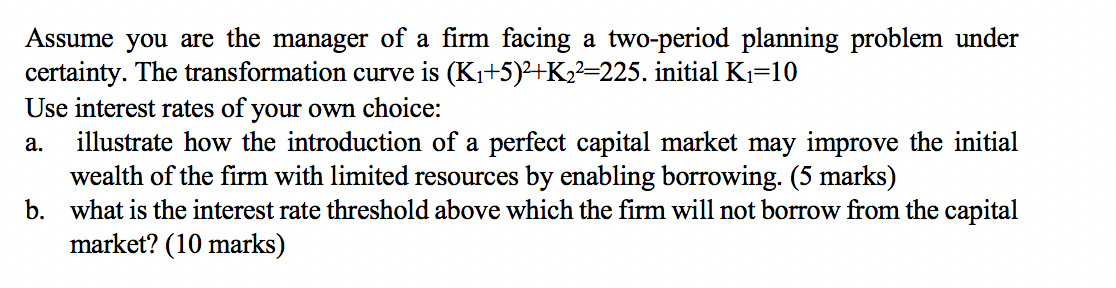### Create an Account

Home / Questions / Assume you are the manager of a firm facing a two-period planning problem under

# Assume you are the manager of a firm facing a two-period planning problem underAssume you are the manager of a firm facing a two-period planning problem under certainty. The transformation curve is (Ki+5)2+K22=225. initial Ki=10 Use interest rates of your own choice: a. illustrate how the introduction of a perfect capital market may improve the initial wealth of the firm with limited resources by enabling borrowing. (5 marks) b. what is the interest rate threshold above which the firm will not borrow from the capital market?

Feb 07 2020 View more View LessGet Solution